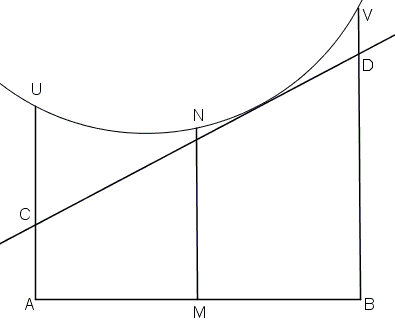Optimization in a Crooked Trapezoid

Figure ABVU consists of three straight line segments and a circular arc. AU||BV, AU⊥AB. Lines tangent to the arc form trapezoids ABDC.Find the tangent that maximizes the area of ABDC.

SolutionFigure ABVU consists of three straight line segments and a circular arc. AU||BV, AU⊥AB. Lines tangent to the arc form trapezoids ABDC.Find the tangent that maximizes the area of ABDC.

The area of any trapezoid ABDC can be found from AB×(AC + BD)/2. In this formula an factor (AB) - the height of the trapezoid - is fixed. The problem then is equivalent to maximizing the half-sum of the bases.In a trapezoid the half-sum of the bases is equal to the midline - the line parallel to the bases and half way between them. Let M be the midpoint of AB, N on the arc such that MN⊥AB. The tangent at N solves the problem. Indeed, all other tangents cross MN below N so that the corresponding midline is shorter than MN.A Sample of Optimization Problems III

• Mathematicians Like to Optimize
• Mathematics in Pizzeria
• The Distance to Look Your Best
• Building a Bridge
• Linear Programming
• Residence at an Optimal Distance
• Distance Between Projections
• Huygens' Problem
• Greatest Difference in Arithmetic Progression
• Area Optimization in Trapezoid
• Minimum under Two Constraints
• Optimization with Many Variables
• Minimum of a Cyclic Sum with Logarithms
• A Problem with a Magical Solution from Secrets in Inequalities
• Leo Giugiuc's Optimization with Constraint
• Problem 4033 from Crux Mathematicorum
• An Unusual Problem by Leo Giugiuc
• A Cyclic Inequality With Constraint in Two Triples of Variables
• Two Problems by Kunihiko Chikaya
• An Inequality and Its Modifications
• A 2-Variable Optimization From a China Competition
•